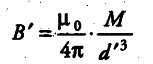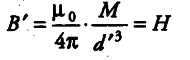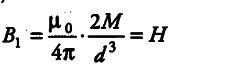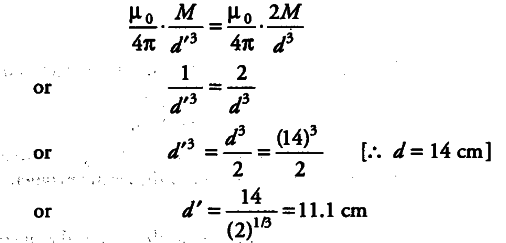# If the bar magnet in Q. 8 is turned around by 180°

If the bar magnet in Q. 8 is turned around by 180°, where will the new null points be located?

When a bar magnet is turned by 180°, then the null points are obtained on the equatorial line.So, magnetic field on the equatorial line at a distance d’ is given byThis magnetic field is equal to’ the horizontal component of the earths magnetic field,-----------------(1)
As we know that, Magnetic field,-----------(2)

From Eqs. (i) and (ii), weThus, the null points are located on the equatorial line at a distance of 11.1 cm.